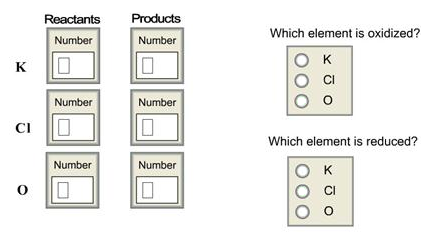# Problem: For the following reaction KClO4 → KCl + 2O2 assign oxidation states to each element on each side of the equation.

###### FREE Expert Solution
97% (482 ratings)
###### FREE Expert Solution
97% (482 ratings)###### Problem Details

For the following reaction KClO4 → KCl + 2Oassign oxidation states to each element on each side of the equation.Frequently Asked Questions

What scientific concept do you need to know in order to solve this problem?

Our tutors have indicated that to solve this problem you will need to apply the Calculate Oxidation Numbers concept. You can view video lessons to learn Calculate Oxidation Numbers. Or if you need more Calculate Oxidation Numbers practice, you can also practice Calculate Oxidation Numbers practice problems.

What is the difficulty of this problem?

Our tutors rated the difficulty ofFor the following reaction KClO4 → KCl + 2O2 assign oxidatio...as medium difficulty.

How long does this problem take to solve?

Our expert Chemistry tutor, Sabrina took 3 minutes and 46 seconds to solve this problem. You can follow their steps in the video explanation above.

What professor is this problem relevant for?

Based on our data, we think this problem is relevant for Professor Courson's class at ODU.